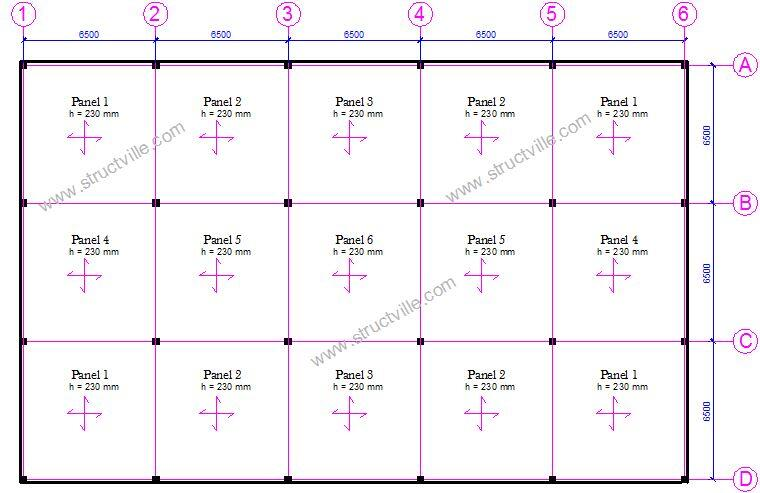# Structural Design of Flat Slabs to Eurocode 2Flat slabs can offer economical solutions to wider floor spans in a reinforced concrete building. Flat slabs are slabs that are supported directly by columns without floor beams. The columns may or may not have drops. There are many advantages of flat slab such as increased head room, easier flow of mechanical and electrical services, ease in construction of form work, faster construction etc.

Flat slabs can be analysed using the frame equivalent method, or by using the simplified analysis method which involves the use of coefficients. On the other hand, finite element analysis using computer programs can be used.  In the Eurocodes, the analysis  of flat slab is the same as that recommended in BS 8110. According to clause 3.7.2.7 of BS 8110, the simplified method can be used for flat slabs that the lateral stability is not dependent on the slab and columns provided that the following conditions are met;

(1) The slab is loaded with a single load case of all the panels loaded with maximum ultimate load. This means that the following requirements must be satisfied (clause 3.5.2.3);
(a) The area of each bay exceeds 30 m2
(c) The imposed load does not exceed 5 kN/m2

(2) There are at least three rows of approximately equal panels in the direction  being considered
(3)  Moments at supports can be reduced by 0.15Fhc

In this post, we are going to analyse panel 6 of the flat slab general arrangement that is given above with the following data;

Thickness of slab = 230 mm (governed by deflection requirements)
fck = 30 Mpa
fyk = 500 Mpa
Concrete cover = 25 mm

Permanent Actions
Self weight = 0.23 × 25 = 5.75 kN/m2
Finishes = 1.2 kN/m2
Partition allowance = 1.5 kN/m2
Total gk = 8.45 kN/m2

Variable Actions
Imposed floor load qk = 4 kN/m2

Total load on the floor F = 1.35gk + 1.5qk = 17.41 kN/m2
F = 17.41 × 6.5 × 6.5 = 735.57 kN

A little consideration will show that the panel under consideration met the conditions for use of coefficients;

For reference on how the panel was divided into middle and column strips, see Figure 3.12 of BS 8110-1:1997.

Bending Moments (In the longitudinal direction)
(1) Centre of the interior span
M = 0.063 × 735.57 × 6.5 = 301.217 kNm

The width of the middle strip is = 6.5 – 1.6 – 1.6 = 3.3 m

Therefore, the middle strip positive moment = 0.45 × 301.217 = 135.547 kNm
The column strip positive moment is given by = 0.55 × 301.217 = 165.669 kNm

(2)  Interior support
M = -0.063 × 735.57 × 6.5 = 301.217 kNm

Therefore, the middle strip moment = 0.25 × 301.217 = 75.304 kNm
The column strip negative moment is given by = 0.75 × 301.217 = 225.912 kNm

Bending Moments (In the lateral direction)
(1) Centre of the interior span
M = 0.063 × 735.57 × 6.5 = 301.217 kNm

The width of the middle strip is = 6.5 – 1.6 – 1.6 = 3.3 m

Therefore, the middle strip positive moment = 0.45 × 301.217 = 135.547 kNm
The column strip positive moment is given by = 0.55 × 301.217 = 165.669 kNm

(2)  Interior support
M = -0.086 × 735.57 × 6.5 = 411.183 kNm

Therefore, the middle strip moment = 0.25 × 411.183 = 102.795 kNm
The column strip negative moment is given by = 0.75 × 411.183 = 308.387 kNm

The reinforcement design is done as usual…

3.Amos